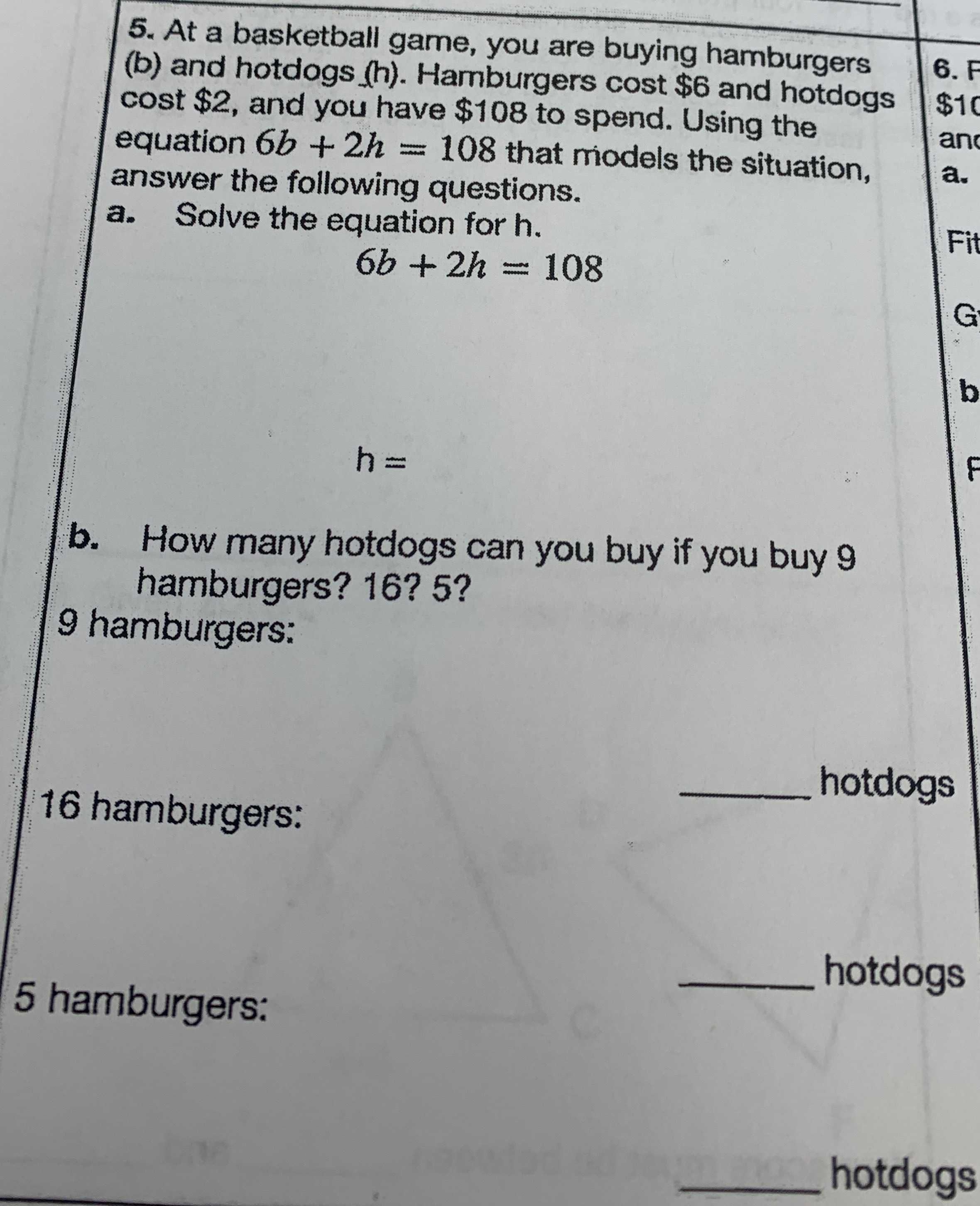### ¿Todavía tienes preguntas de matemáticas?

Pregunte a nuestros tutores expertos
Algebra
Pregunta5. At a basketball game, you are buying hamburgers (b) and hotdogs (h). Hamburgers cost $$\ 6$$ and hotdogs cost $$\ 2$$ , and you have $$\ 108$$ to spend. Using the equation $$6 b + 2 h = 108$$ that miodels the situation, answer the following questions. a. Solve the equation for $$h$$ .

$$6 b + 2 h = 108$$ $$h =$$ b. How many hotdogs can you buy if you buy $$9$$ hamburgers? 16? $$5$$ ?

$$9$$ hamburgers: $$16$$ hamburgers: $$\quad$$ hotdogs $$5$$ hamburgers: $$\quad$$ hotdogs